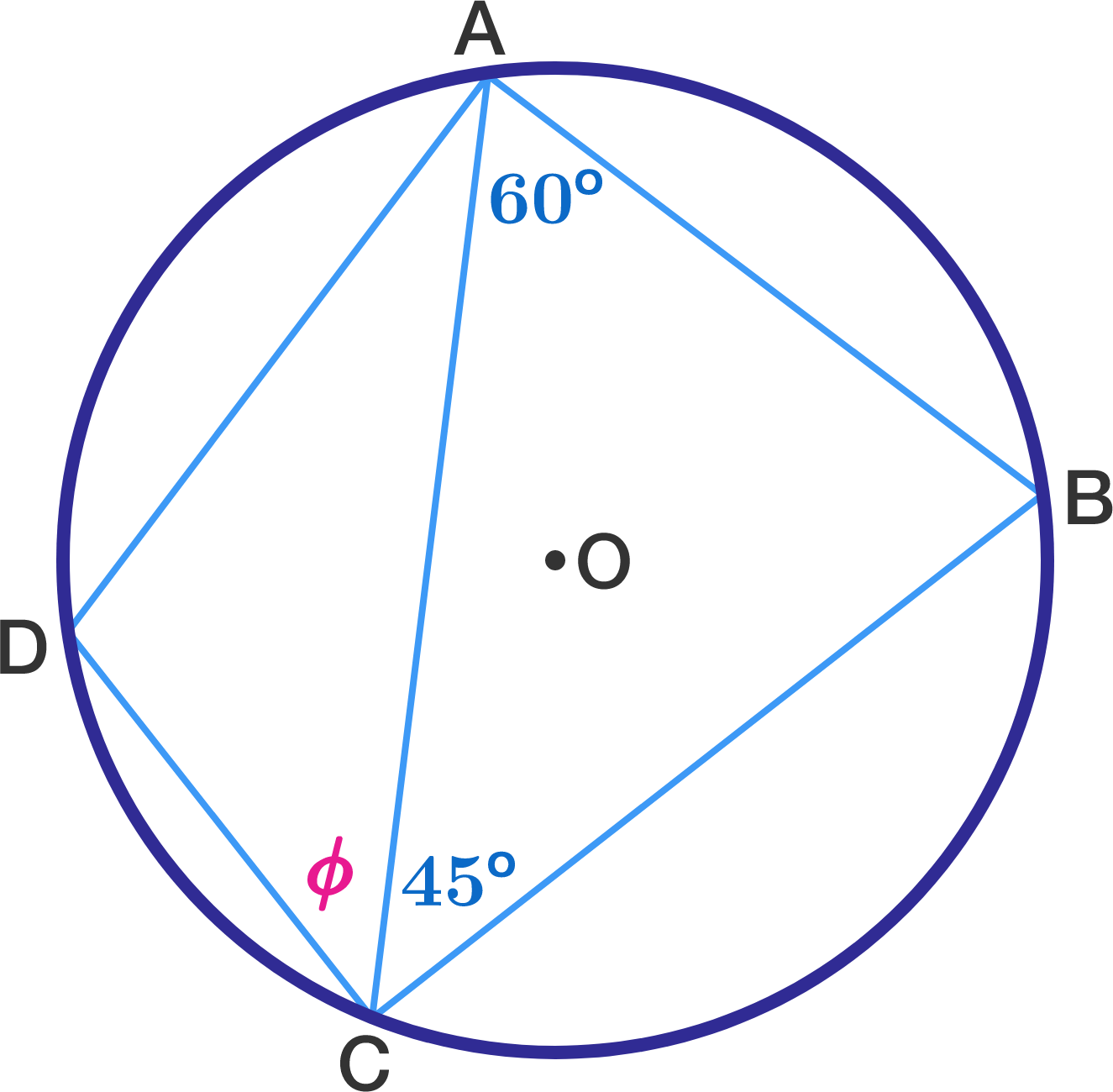Geometry Level 1The circle above has its center at point $O$, and arc $DC$ is half the length of arc $BC$.

What is the measure of angle $\phi?$

×